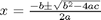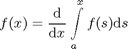# LaTeX Formula Publishing

A long time ago the Publish team added the ability to include LaTeX formulas in published MATLAB files. This was seen as a nice way to include pretty-formatted formulas and equations without having to resort to ASCII art. In order to make sure the published formulas are cross-browser compatible, the generated formulas are included into the published document as images.

New in MATLAB R2011a is the ability to include inline LaTeX formulas. Previously they were only available in their own block. An inline LaTeX formula is surrounded by single “$“‘s, and a block is separated by double “$$“‘s. Sample markup can also be added using the top-level Cell Menu: “Cell -> Insert Text Markup -> LaTeX Inline Math” for inline markup and “Cell > Insert Text Markup > LaTeX Display Math” for block markup. In this example I’ve included both Inline and Display Block LaTeX formulas: % % Some people's favorite equation is the quadratic formula: x = \frac{-b % \pm \sqrt{b^2 - 4ac}}{2a}. But some people prefer the Fundamental Theorem % of Calculus: % %$$f(x) = {\mathrm{d} \over \mathrm{d}x} \int\limits_a^x f(s)\mathrm{d}s$\$
%

Here are the published results:

Some people’s favorite equation is the quadratic formula:. But some people prefer the Fundamental Theorem of Calculus:|